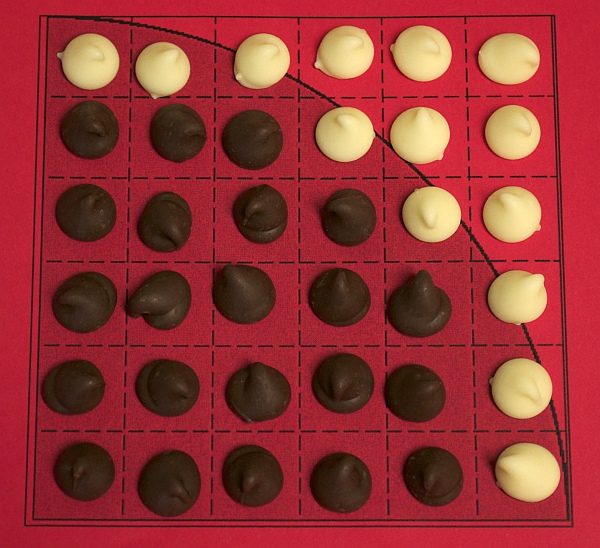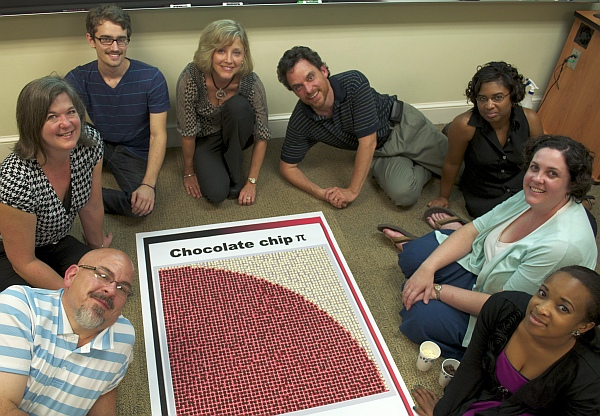### Math Monday: Candy Pi Calculator

by George Hart

There are many ways to calculate an approximation to pi, but rarely is math as delicious as in this idea from Davidson College professor Tim Chartier. Make a quarter circle in a square of graph paper and place chocolate chips on the squares that lie completely inside the circle. If you now count the chips and compute four times the number of chocolate chips divided by the total number of squares, that will be approximately pi. Here, there are 22 chips out of 36 squares, so we calculate 4·22/36=2.444, which is off by about 0.7 from 3.14, so is not a very close estimate of pi, but we can improve it.If you are willing to invest in more chocolate, you will get a closer answer. In the 11×11 grid below, you can count 83 chips, giving a somewhat better approximation of 4·83/121=2.74.There are other approximation techniques in which the squares that the circle passes through are also counted. But for better and better estimates, you’ll want to work in a finer grid with a group of helpers. Tim and friends counted 2232 chips in a 54×54 grid, resulting in an approximation of 3.06, which is getting pretty close to pi. The best thing about this chocolate calculation is that it is sure to end with a pi eating event. The full report is here.